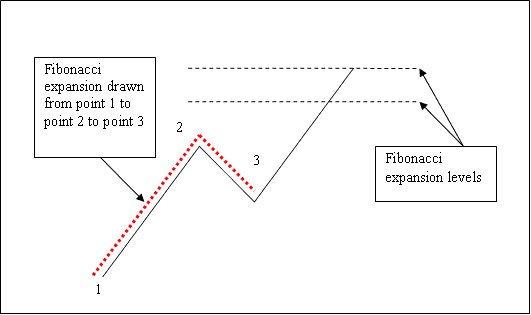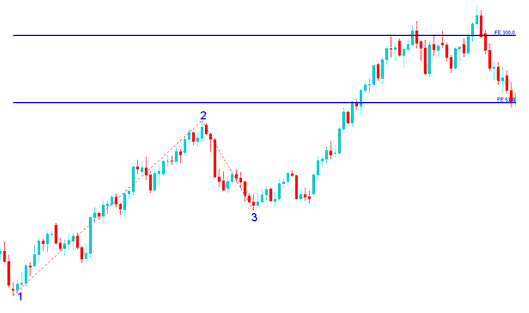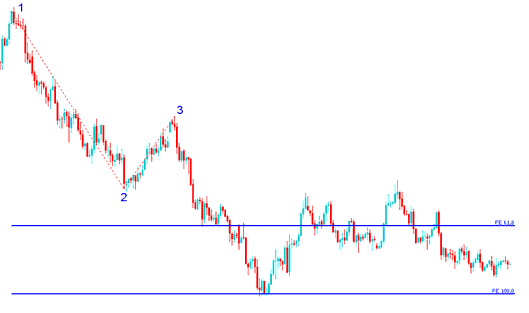Learn Gold Trading for Beginners Tutorials

# XAUUSD Fibonacci Expansion Levels

We have looked at Fibonacci retracement in the previous lesson, drawn between 2 points. But to draw Fibonacci expansion we use 3 chart points.

To draw these levels we wait until the retracement is complete and the xauusd price starts to move in the original direction of the XAUUSD trend. Where the retracement reaches is used as point 3.

The example shown below shows the 3 Points where the Fibonacci extension is drawn, marked as 1, 2 and 3. 1 is where the xauusd trend started, 2 is where the xauusd trend pulled back and retraced and 3 is where the retracement reached as shown on the xauusd example shown below.Please note where these levels are drawn - they are drawn above the indicator, these are the points where the xauusd trader will set the take profit orders.

### Drawing Expansion Levels on an Upward Trend

We use Fibonacci extension levels to estimate where the movement will reach. There are 2 important extension levels; 61.8 % and 100 %, these are used for taking profit.

On the example, below you can see that the Fibonacci extension is drawn along the direction of the trend, since the xauusd trend is upward - the expansion is drawn upwards.

These levels are displayed as horizontal lines above the indicator, showing profit taking areas. In the xauusd example shown below if you had used of 100 % extension you would have made nice profit from the trade.

NB: This is the same trade from the previous example where we used Fibonacci retracement to buy at around retracement level 38.2 %. At the same time we have used Fibonacci expansion 100 % to set take profit. Now find a XAUUSD Trading chart and practice these strategies.From the above examples, the upward xauusd trend continued and both 61.8 % and 100.0 % levels were all hit after which gold price retraced again after hitting the 100.0 % extension.

### Drawing Expansion Levels on a Downward Trend

Since we use this tool to estimate take profits, how do we draw it in a downward XAUUSD trend?

We draw it from point 1 to 2 to 3 as shown below. Remember we always draw this tool in the direction of the trend. In the xauusd example shown below, can you figure what direction we have drawn it? That is right - downwards.

Try and see the difference between how we have drawn it above and how it is drawn below. This time you would also have used extension level 100 %, see just where the gold price reached. That would have been a nice take profit area.From the xauusd example above, after plotting this tool there are two levels that are used to show the profit taking areas, these two are drawn as horizontal lines across the gold price chart.